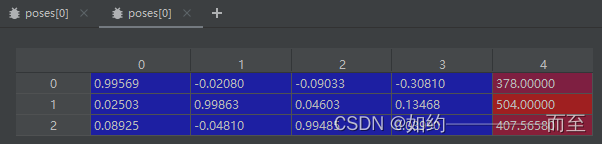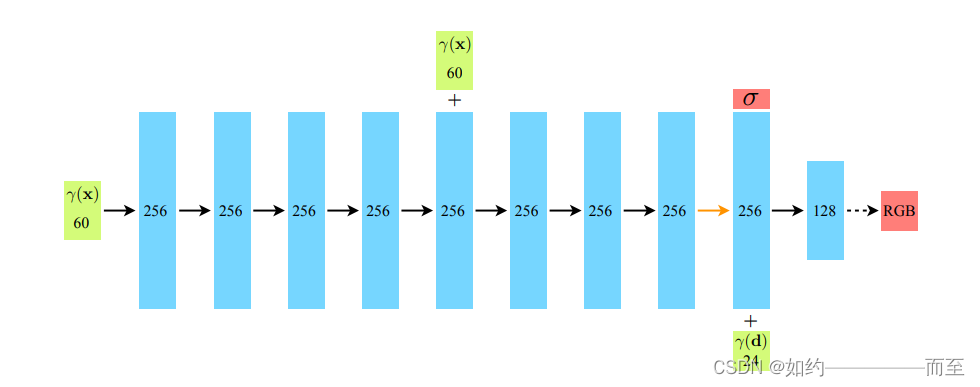# 程序员最近都爱上了这个网站  程序员们快来瞅瞅吧！  it98k网:it98k.com

出租广告位,需要合作请联系站长

+关注

## Pytroch Nerf代码阅读笔记（LLFF 数据集pose 处理和Nerf 网络结构）

`````` images, poses, bds, render_poses, i_test = load_llff_data(args.datadir, args.factor,
recenter=True, bd_factor=.75,
spherify=args.spherify)
``````pose 蓝色部分包含rotation matrix 和 translation vector，就是平移和旋转，是一般意义上的位姿矩阵T （camera-to-world affine）。 第4列红色的部分，分别代表图像的高height,宽度width，和相机的焦距Focal:在train函数里面有如下代码：

``````hwf = poses[0,:3,-1]  // 取出前三行最后一列元素（红色部分）
poses = poses[:,:3,:4]  // 取出pose里的平移和旋转部分
....中间代码略去.......
H, W, focal = hwf   // 分别赋予 Hieight、Width、focal
``````

## Nerf 代码的阅读：

#### Nerf 网络的搭建：Input: layer = 0,Position Encoding 后的长度为 63 的vector
layer =9 时，将第8层的输出（channel=256）和 direction 进行Postion Encoding 之后（channel=27）进行concat

Output: 第8层的 density 为 alpha 的输出 和第10层的 rgb 3channel 的输出

netdepth = 8 , netwidth = 256 , input_ch = 63，是指position输入的维度（position encoding 之后的编码），skip = 4， 是因为在论文中 第5层出现了 skip connection.

``````model = NeRF(D=args.netdepth, W=args.netwidth,
input_ch=input_ch, output_ch=output_ch, skips=skips,
input_ch_views=input_ch_views, use_viewdirs=args.use_viewdirs).to(device)
``````

Nerf 的 网络构建代码如下：

``````class NeRF(nn.Module):
def __init__(self, D=8, W=256, input_ch=3, input_ch_views=3, output_ch=4, skips=, use_viewdirs=False):
"""
"""
super(NeRF, self).__init__()
self.D = D
self.W = W
## Position Encoding之后的 位置vector通道数（63）
self.input_ch = input_ch
## Position Encoding之后的 direction的vector通道数（27）
self.input_ch_views = input_ch_views
self.skips = skips   ## 在第4层有跳跃连接
self.use_viewdirs = use_viewdirs

## 前8层的MLP实现：输入为63，输出为 256
self.pts_linears = nn.ModuleList(
[nn.Linear(input_ch, W)] + [nn.Linear(W, W) if i not in self.skips else nn.Linear(W + input_ch, W) for i in range(D-1)])

### 构建了第9层的输入为 第8层的输出 和 direction 进行concat,输出为128 维
self.views_linears = nn.ModuleList([nn.Linear(input_ch_views + W, W//2)])

if use_viewdirs:
self.feature_linear = nn.Linear(W, W) # 第9层 输出256维的向量
self.alpha_linear = nn.Linear(W, 1) # 第9层输出 density alpha(1维)
self.rgb_linear = nn.Linear(W//2, 3)
else:
self.output_linear = nn.Linear(W, output_ch)

def forward(self, x):
input_pts, input_views = torch.split(x, [self.input_ch, self.input_ch_views], dim=-1)
h = input_pts
for i, l in enumerate(self.pts_linears):
h = self.pts_linears[i](h)
h = F.relu(h)
if i in self.skips:
h = torch.cat([input_pts, h], -1)

if self.use_viewdirs:
alpha = self.alpha_linear(h)
feature = self.feature_linear(h)
h = torch.cat([feature, input_views], -1) #第9层concat direction 向量

for i, l in enumerate(self.views_linears):
h = self.views_linears[i](h)
h = F.relu(h)

rgb = self.rgb_linear(h)  ## 输出rgb 3维度向量
outputs = torch.cat([rgb, alpha], -1)
else:
outputs = self.output_linear(h)

return outputs
``````

2 0

pdf(new) 更多>